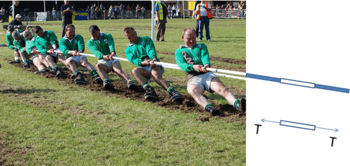# Tension (physics)In physics, tension describes the pulling force transmitted axially by means of a string, cable, chain, or similar one-dimensional continuous object, or by each end of a rod, truss member, or similar three-dimensional object; tension can also be described as the action-reaction pair of forces acting at each end of said elements. Tension is the opposite of compression.

At the atomic level, when atoms or molecules are pulled apart from each other and gain potential energy with a restoring force still existing, the restoring force creates what is also called tension. Each end of a string or rod under such tension will pull on the object it is attached to, to restore the string/rod to its relaxed length.

In physics, tension, as a transmitted force, as an action-reaction pair of forces, or as a restoring force, is a force and has the units of force measured in newtons (or sometimes pounds-force). The ends of a string or other object transmitting tension will exert forces on the objects to which the string or rod is connected, in the direction of the string at the point of attachment. These forces due to tension are also called "passive forces". There are two basic possibilities for systems of objects held by strings: either acceleration is zero and the system is therefore in equilibrium, or there is acceleration, and therefore a net force is present in the system.

## Tension in one-dimensional continueTension in a string is a non-negative scalar quantity. Zero tension is slack. A string or rope is often idealized as one dimension, having length but being massless with zero cross section. If there are no bends in the string, as occur with vibrations or pulleys, then tension is a constant along the string, equal to the magnitude of the forces applied by the ends of the string. By Newton's Third Law, these are the same forces exerted on the ends of the string by the objects to which the ends are attached. If the string curves around one or more pulleys, it will still have constant tension along its length in the idealized situation that the pulleys are massless and frictionless. A vibrating string vibrates with a set of frequencies that depend on the string's tension. These frequencies can be derived from Newton's laws of motion. Each microscopic segment of the string pulls on and is pulled upon by its neighboring segments, with a force equal to the tension at that position along the string. tensionwhereis the position along the string.

If the string has curvature, then the two pulls on a segment by its two neighbors will not add to zero, and there will be a net force on that segment of the string, causing an acceleration. This net force is a restoring force, and the motion of the string can include transverse waves that solve the equation central to Sturm-Liouville theory:whereis the force constant per unit length [units force per area]are the eigenvalues for resonances of transverse displacementon the string., with solutions that include the various harmonics on a stringed instrument.

## Tension in three-dimensional continue

Tension is also used to describe the force exerted by the ends of a three-dimensional, continuous material such as a rod or truss member. Such a rod elongates under tension. The amount of elongation and the load that will cause failure both depend on the force per cross-sectional area rather than the force alone, so stress = axial force / cross sectional area is more useful for engineering purposes than tension. Stress is a 3x3 matrix called a tensor, and theelement of the stress tensor is tensile force per area, or compression force per area, denoted as a negative number for this element, if the rod is being compressed rather than elongated.

## System in equilibrium

A system is in equilibrium when the sum of all forces is zero.For example, consider a system consisting of an object that is being lowered vertically by a string with tension, T, at a constant velocity. The system has a constant velocity and is therefore in equilibrium because the tension in the string, which is pulling up on the object, is equal to the weight force, mg ("m" is mass, "g" is the acceleration caused by the gravity of Earth), which is pulling down on the object.## System under net force

A system has a net force when an unbalanced force is exerted on it, in other words the sum of all forces is not zero. Acceleration and net force always exist together.For example, consider the same system as above but suppose the object is now being lowered with an increasing velocity downwards (positive acceleration) therefore there exists a net force somewhere in the system. In this case, negative acceleration would indicate that.In another example, suppose that two bodies A and B having massesand, respectively, are connected with each other by an inextensible string over a frictionless pulley. There are two forces acting on the body A: its weight () pulling down, and the tensionin the string pulling up. Therefore, the net forceon body A is, so. In an extensible string, Hooke's law applies.

## Strings in modern physics

String-like objects in relativistic theories, such as the strings used in some models of interactions between quarks, or those used in the modern string theory, also possess tension. These strings are analyzed in terms of their world sheet, and the energy is then typically proportional to the length of the string. As a result, the tension in such strings is independent of the amount of stretching.Wikimedia Commons has media related to Tension.